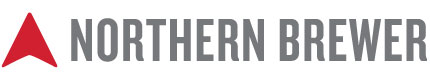# EZ Brew 1.95 Is Now Available

[attachment=0]EZ Brew 1.95.zip[/attachment]

EZ Brew 1.95 is now available.

Added Danstar Abbaye Yeast to yeast strains sheet and drop down list.
Added 14 yeast / sour strains from The Yeast Bay to yeast strains sheet and drop down list.
Added 23 yeast / sour strains from East Coast Yeast to yeast strains sheet and drop down list.
Corrected the formula in cell L66, Pre-boil Liquid Volume.

New formula =IF(I29=1,0,ROUND((D9+L11+L14)/(1-D12/100)/(1-D11/100D10/60),2))
Previous formula =IF(I29=1,0,ROUND((D9+L11+L14)
(1+D12/100)(1+D11/100D10/60),1))
Corrected the formula in cell D67, Total Water Volume.
New formula =IF(I29=1,0,ROUND((D9+L11+L14)/(1-D12/100)/(1-D11/100D10/60)+L12+L13+L62,2))
Previous formula =IF(I29=1,0,ROUND((D9+L11+L14)
(1+D12/100)(1+D11/100D10/60)+L12+L13+L62,2))
Corrected the formula in cell D79, Campden Tablets Strike Water Quantity to account for % distilled water used.
New formula =ROUND(((1/60D65)(1-‘EZ Water 3.0’!D9)),2)
Previous formula =ROUND(1/60D65,2)

Corrected the formula in cell E79, Campden Tablets Strike Water Quantity to account for % distilled water used.
New formula =ROUND(((1/15D6^)(1-‘EZ Water 3.0’!E9)),2)
Previous formula =ROUND(1/15
D66,2)

Deleted Hop Inventory tab.
Deleted Grain Inventory tab.

Please let me know if you find anything wrong with the spreadsheet so I can update it.
Thank you,
Gregscsu